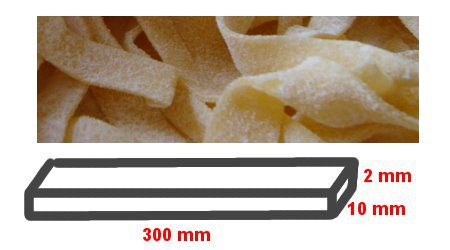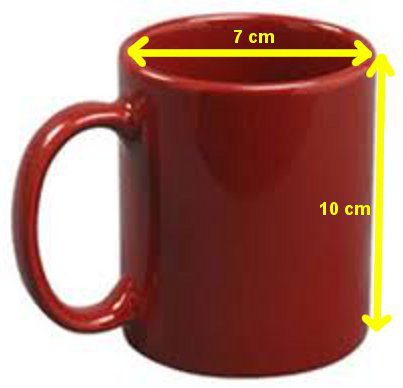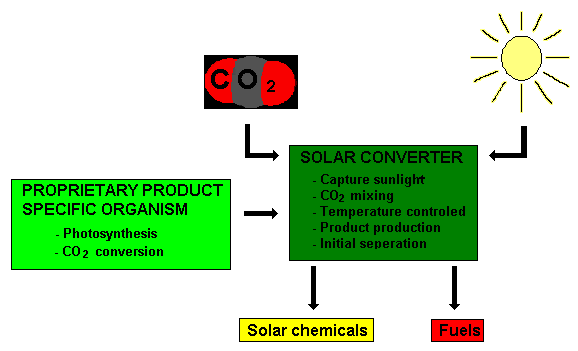# Volume Of Prisms

Prisms are 3-dimensional solids that have the same cross-section (the shape of its base or end) all the way through. For example, a rectangular prism has the same rectangle shape at both ends; a triangular prism has the same triangle shape at both ends; and a cylinder (a circle-based prism) has the same circle shape at both ends.

Volume is the amount of solid inside an object and is measured in cubic units (e.g. cubic metres and cubic centimetres).

Capacity is the amount of gas or liquid inside an object and is measured in litres and millilitres.

The general rule to calculate the volume of a prism is:

Volume of Prism = Area of Cross-section × Height

To convert from volume to capacity, it is important to know that:

• 1 cubic centimetre = 1 millilitre
• 1 cubic metre = 1 kilolitre

Examples of Volume and Capacity are:

• rock in a quarry
• plastic in a plumbing pipe
• concrete slabs
• air in a room (air-conditioning)
• gas in a barbecue gas bottle
• gasoline (petrol) in a car's tank
• milk in a carton
• water in a rainwater tank or a swimming pool

To change:

• cubic centimetres (cm3) to cubic millimetres (mm3), multiply by 10 and again by 10 and again by 10 (or multiply by 1000).
• cubic metres (m3) to cubic millimetres (mm3), multiply by 1000 and by 1000 again and by 100 again (or multiply by 1 000 000 000).
• cubic metres (m3) to cubic centimetres (cm3 or cc) , multiply by 100 and by 100 again and by 100 again (or multiply by 1 000 000).
• cubic kilometres (km3) to cubic metres (m3), multiply by 1000 and by 1000 again and by 1000 again (or multiply by 1 000 000 000).

IF YOU CUBE THE NAME, YOU CUBE THE NUMBER.

## Example One - FettuccineFettuccine is pasta with a rectangular prism shape. It is like flat spaghetti. Each piece is 10 mm wide, 300 mm long and 2 mm thick. What is amount of pasta needed to make one piece?

The cross-section of a rectangular prism is a rectangle.
Take care not to mix up the height measurements.

Volume of Prism
= Area of Cross-section × Height
= Area of Rectangle × Height
= (L × H) × Height
= (10 × 2) × 300
= 6000 mm3 of fettuccine

## Challenge Question - Optimal Size of a Take-Away Box

A restaurant's take-away box (with lid) is to be designed to hold 600 cubic centimetres of food. A rectangular prism is easier to store. The smallest surface area would mean less material and therefore lower cost. What are the optimal (best) dimensions for this box?

## Example Two - Coffee MugA coffee mug is a circle-based prism or cylinder shape.

This mug's diameter is 7 cm. The height is 10 cm. What is the capacity of coffee in a full mug? (Use π = 3.14.)

The cross-section of a cylinder is a circle.

Volume of Prism
= Area of Cross-section × Height
= Area of Circle × Height
= π r2 × H
= 3.14 × 3.5 × 3.5 × 10
= 384.65 cm3
= 384.65 millilitres of coffee

## Example Three - MacaroniEach piece of macaroni has a larger diameter of 5 mm and a small diameter of 3 mm. The length of each piece is 10 mm. What volume of pasta is needed to make one piece?
(Use π = 3.14.)

The cross-section of macaroni is an annulus (a large circle minus a small circle).

Area of Cross-section
Area of large circle
= π R2
= 3.14 × 5 × 5
78.5 mm2

Area of small circle
= π r2
= 3.14 × 3 × 3
28.26 mm2

Area of annulus
= 78.5 – 28.26
=50.24 mm2

Volume
= Area of Cross-section × Height
= 50.24 × 10
= 502.4 mm3 of macaroni

## Question - C-R-A-Z-Y Classroom Capacity

1. What are your classroom dimensions - length, width and height? Write these in both metres and centimetres.
2. Calculate the room's volume in both cubic metres and cubic centimetres.
3. How much water would it take to completely fill the room? Remember that 1 cubic centimetre equals 1 millilitre. Now work this out in litres and kilolitres also.
4. Calculate how many cans of 375 ml cola it would take to fill the room.
5. Presuming that the volume of your body is 60 000 ml, calculate how much water/cola it would take to fill the room if you were in the room. There is no furniture.
6. In order to stay alive and not drown, you must drink enough water/cola to create a 10 cm air space at the top of the room. Calculate how much you need to drink. (Imagine that your bladder has an infinite capacity so it's not coming out the other end as you drink! Also, assume that your body is not swelling as you drink and you can drink really fast!!)
7. If you work this out correctly, you stay alive.

(Would it have been easier to break the window?)

## Did You Know That...?Helioculture is the process to make hydrocarbon-based fuel from genetically modified photosynthesizing bacteria from waste water (from baths and washing machines), carbon dioxide, nutrients and sunlight. According to manufacturer "Joule Unlimited", this alternative to crude oil can be produced at 19000 cubic metres per square kilometre and will be cost-effective when crude oil costs US\$50 per barrel (or \$310 per cubic metre). Currently, crude oil costs about US\$95 per barrel.